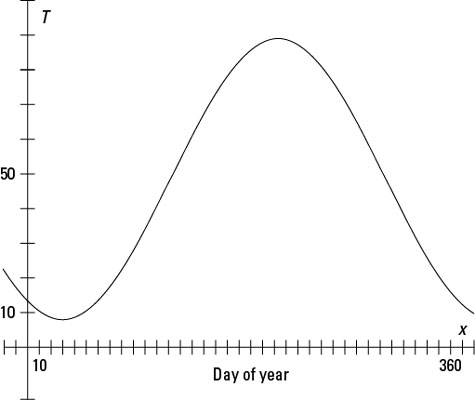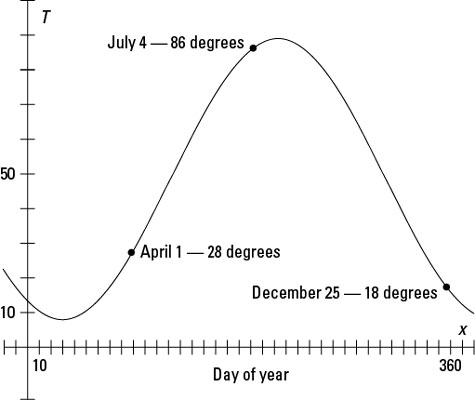##### Trigonometry For DummiesYou can use the graph for a trigonometry function to show average daily temperatures at a specific location. For example, a relatively reasonable model for the average daily temperature in Peoria, Illinois, is T(x) = 50 – 42 cos (0.017x – 0.534), where x is the day of the year starting with January 1 as Day 1. The T(x) represents the temperatures in degrees Fahrenheit. The graph is in radian measure, because radians are real numbers, as opposed to degrees — and that’s how you count off the days. The following figure shows what the graph of the function looks like for the whole year.The average daily temperature in Peoria, Illinois, on Day x.

The multiplier on the cosine function is 42, so the amplitude of the curve is 42. Don’t worry about the negative sign in front of the 42. The curve goes upward and downward anyway, so the negative sign just makes it go downward and then upward, instead of the reverse, which represents those cold days in January.

The period is affected by the multiplier 0.017. The result of that multiplication is that only 0.017 of a cosine curve takes up the usual amount of space for an entire curve, which is 2π, or a little over 6 units. Because this graph is for a whole year, the curve has to spread out over 365 units, so that each of the horizontal units has just a little part of it.

The shift upward of 50 units is the middle temperature for the year. Add the amplitude of 42 to this number, and the average temperature gets up to 92 degrees; subtract the amplitude, and the average gets down to 8 degrees. Note that the curve starts a little to the right of the y-axis to account for when the seasons change.Dates and average temperatures in Peoria, Illinois.

What do you do with the graph? You can estimate when the highest and lowest temperatures occur and get an idea of the types of temperatures to expect if you move to Peoria, Illinois. The preceding figure shows the graph of the average temperatures with points for some days of the year and the average temperatures on those days. A graphing calculator is indispensable when graphing these figures and calculating values. You can either input x-values to find out what the y-values are at those points, or you can trace along the curve to get the measures.